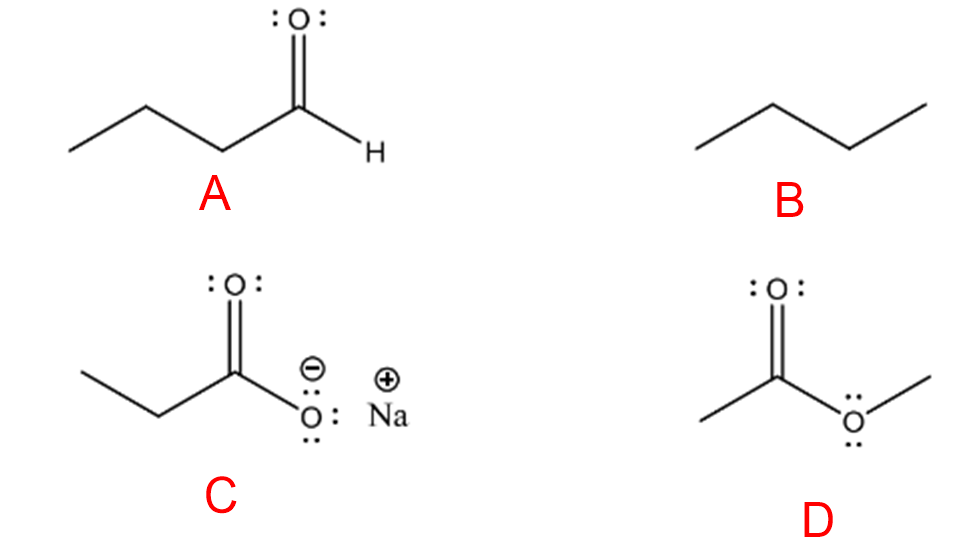# Problem: For each pair, circle the chemical species that you would expect to be the most soluble in water.

82% (99 ratings)
###### FREE Expert Solution

We’re being asked to determine the compound in each pair that would be more soluble in water.

Recall that the main idea in dissolution is like dissolves like, which means compounds with the same polarity and intermolecular force can dissolve each other.

Water is a polar molecule that exhibits hydrogen bonding, so the best solute is another polar compound. For this problem, we need to determine the polarity of each molecule.

82% (99 ratings)###### Problem Details

For each pair, circle the chemical species that you would expect to be the most soluble in water.Frequently Asked Questions

What scientific concept do you need to know in order to solve this problem?

Our tutors have indicated that to solve this problem you will need to apply the How To Determine Solubility concept. You can view video lessons to learn How To Determine Solubility. Or if you need more How To Determine Solubility practice, you can also practice How To Determine Solubility practice problems.